Full Text:<2412>

CLC number: TU3

On-line Access: 2013-03-04

Revision Accepted: 2012-08-02

Crosschecked: 2013-02-22

Cited: 5

Clicked: 4410

Citations:  Bibtex RefMan EndNote GB/T7714

 Journal of Zhejiang University SCIENCE A 2013 Vol.14 No.3 P.206-218 http://doi.org/10.1631/jzus.A1200114

Characteristics of bifurcation and buckling load of space truss in consideration of initial imperfection and load mode*

 Author(s):  Su-deok Shon1, Seung-jae Lee1, Kang-guk Lee2 Affiliation(s):  1. School of Architectural Engineering, Korea University of Technology and Education, 1800 Chungjeolro, Cheonan Chungnam, Republic of Korea; more Corresponding email(s):   leeseung@koreatech.ac.kr Key Words:  Space truss, Geometric nonlinearity, Initial imperfection, Snap-through, Bifurcation, Global buckling Share this article to： More <<< Previous Article|Next Article >>>

Su-deok Shon, Seung-jae Lee, Kang-guk Lee. Characteristics of bifurcation and buckling load of space truss in consideration of initial imperfection and load mode[J]. Journal of Zhejiang University Science A, 2013, 14(3): 206-218.

@article{title="Characteristics of bifurcation and buckling load of space truss in consideration of initial imperfection and load mode",
author="Su-deok Shon, Seung-jae Lee, Kang-guk Lee",
journal="Journal of Zhejiang University Science A",
volume="14",
number="3",
pages="206-218",
year="2013",
publisher="Zhejiang University Press & Springer",
doi="10.1631/jzus.A1200114"
}

%0 Journal Article
%T Characteristics of bifurcation and buckling load of space truss in consideration of initial imperfection and load mode
%A Su-deok Shon
%A Seung-jae Lee
%A Kang-guk Lee
%J Journal of Zhejiang University SCIENCE A
%V 14
%N 3
%P 206-218
%@ 1673-565X
%D 2013
%I Zhejiang University Press & Springer
%DOI 10.1631/jzus.A1200114

TY - JOUR
T1 - Characteristics of bifurcation and buckling load of space truss in consideration of initial imperfection and load mode
A1 - Su-deok Shon
A1 - Seung-jae Lee
A1 - Kang-guk Lee
J0 - Journal of Zhejiang University Science A
VL - 14
IS - 3
SP - 206
EP - 218
%@ 1673-565X
Y1 - 2013
PB - Zhejiang University Press & Springer
ER -
DOI - 10.1631/jzus.A1200114

Abstract:
This study investigated characteristics of bifurcation and critical buckling load by shape imperfection of space truss, which were sensitive to initial conditions. The critical point and buckling load were computed by the analysis of the eigenvalues and determinants of the tangential stiffness matrix. The two-free-nodes example and star dome were selected for the case study in order to examine the nodal buckling and global buckling by the sensitivity to the eigen buckling mode and the analyses of the influence, and characteristics of the parameters as defined by the load ratio of the center node and surrounding node, as well as rise-span ratio were performed. The sensitivity to the imperfection of the initial shape of the two-free-nodes example, which occurs due to snapping at the critical point, resulted in bifurcation before the limit point due to the buckling mode, and the buckling load was reduced by the increase in the amount of imperfection. The two sensitive buckling patterns of the numerical model are established by investigating the displaced position of the free nodes, and the asymmetric eigenmode greatly influenced the behavior of the imperfection shape whether it was at limit point or bifurcation. Furthermore, the sensitive mode of the two-free-nodes example was similar to the in-extensional basis mechanism of a simplified model. The star dome, which was used to examine the influence among several nodes, indicated that the influence of nodal buckling was greater than that of global buckling as the rise-span ratio was higher. Besides, global buckling is occurred with reaching bifurcation point as the value of load ratio was higher, and the buckling load level was about 50%–70% of load level at limit point.

## 1.  Introduction

Space truss, which belongs to such light structure category as continuous shell, membrane, cable net, etc., has the advantage of being relatively small in weight and enables long span in form-activity shape to transfer the force through in-plane stress. This space truss resembles continuous shell in force flow and has many mechanical advantages as well as aesthetic appearance, but it has a structural instability problem in the shell, which must be dealt with. In other words, if a long span is made thin, by the structural principle of shell or arch, such unstable phenomenon as snap-through and bifurcation buckling takes place due to geometrical non-linearity, and it is very sensitive to the initial condition (Thompson and Hunt, ; El-Sheikh,; Mang et al., ; Lopez et al., ; Yamada et al., ). The sensitivity to initial imperfection exerts ultimate influence on the progression from primary path to bifurcation path, and characteristics of the bifurcation due to imperfection of the shape or load parameter were one of the favorite research topics. The critical point and bifurcation existing on the equilibrium path is affected by a complex influence of parameters stemming from imperfection. Huseyin () investigated an extended perturbation technique to solve this multi-parameter stability problem. The method of bifurcation analysis is discussed in (Choong and Hangai, ) in detail, and the analysis dealt with the prediction of direct and indirect bifurcations (Abedi and Parke, ) as well as the methods using generalized inverse for geometrical non-linearity within elastic range of the conservative system. Choong and Kim () suggested a method to find the stability boundaries for a simple critical point with multi-parameters. Generally, the critical point can be determined by the characteristics of the determinants, eigenvalue and eigenvector of the tangential stiffness matrix (Shon et al., ; You et al., ).

The space truss with curvature has instability problem mainly due to a complex combination of member buckling, nodal buckling and global buckling of the whole structure (Bulenda and Knippers, ; Lopez et al., ; Kato et al., ). In this regard, Kato et al. () investigated the influence of nodal rigidity on the buckling stress of a single-layer lattice dome. Moreover, Chan and Zhou () developed a second-order elastic analysis in consideration of the initial imperfection of each member and derived the stiffness matrix in consideration of imperfection. While their studies focused on the member buckling stress of a structure in consideration of the member buckling, Bulenda and Knipper () conducted a study on parameters for the eigen buckling mode of a grid shell structure and investigated the influence of initial imperfect shape and its application.

A lot of research has been carried out on stability (Shon et al., ; Hwang and Knippers, ; You et al., ; Uros et al., ), buckling load (Kato et al., ; Fan et al., ; Yamada et al., ) and various joint rigidities (Ma et al., ; Fan et al., ) in consideration of the initial imperfection of a shell-type space truss. Also, the geometrical nonlinearity, the post-buckling and qualitative improvement of sensitive structures (Gao et al., ; Schranz et al., ; Steinboeck et al., ) have become more and more attractive. Shon et al. () analyzed the effect of global instability caused by joint rigidity, and Lopez et al. () carried out analytic and experimental research into the Euler buckling of a member and snap-through at the node. Besides, Fan et al. () attempted to discover the buckling load in consideration of the initial bending of a member. The unstable behavior characteristics and critical load are studied according to various parameters because they are very important in the design of the space truss, which is sensitive to the initial conditions. The space truss has very complex characteristics depending on the shape or load conditions.

In this study, the characteristics of the critical load due to the generation of a bifurcation path and point were investigated, according to the initial imperfection of the space truss, for a shallow space truss and a star dome. The bifurcation path was analyzed based on its imperfect shapes, by eigenmode. The characteristics of sensitivity and critical load due to the bifurcation were also investigated. In particular, the characteristics of the critical loads for the global buckling and for the bifurcation point of the star dome and nodal buckling in the equilibrium path were studied in accordance with the rise-span and nodal-load ratios.

## 2.  Identification of critical point and bifurcation for space truss

For the study of the bifurcation behavior and critical load of a space truss, geometric nonlinearity needs to be considered in the derivation of an equation in the elastic domain. To derive incremental stiffness equations considering the nonlinear term, a displacement function was assumed using translational displacement d at the node. , where Ni (=1−x/l) and Nj (=x/l) are the shape functions, and I n is the unit matrix (n-dimension), n=3.

Geometrical nonlinearity can be considered by including the second term of the strain-displacement relationship based on the assumption of Bernoulli-Euler. , where , where εx is the axial strain, and (,x) is partial differential with respect to x, i.e., .

The equation based on the current state using the principle of virtual work is given as , where σx (0) is the axial stress at previous step, and f is the nodal force vector (local).

If δεx in Eq. (2) is determined and substituted in Eq. (3), the following equation is obtained: , where A and l are the sectional area and length of the member, respectively.

If only the elastic domain of the material is considered, Eq. (4) can be rewritten as follows, using the relation σx=Eεx , , where E is the elastic modulus.

If the higher-order term is omitted and the resultant error is defined as residual force r , the following tangential stiffness matrix and incremental equations is obtained: , where .

If the coordinate transformation matrix T is used to express the stiffness matrix with global coordinates, we can obtained: , where the first term represents elastic stiffness matrix, and the second term denotes geometrical stiffness matrix.

Nonlinear incremental analysis can be performed using the above matrix. The determinant and eigenvalues at each step can be provided with information about the unstable phenomenon in the equilibrium path.

The method of identifying the critical point by using the determinant and eigenvalue in each incremental region on a nonlinear primary path is most commonly used. The incremental equations of the global coordinates expressed as the matrix in Eq. (7) can be simplified as follows: , where F is the nodal force vector (global), λ is the load parameter, D is the nodal displacement vector (global). The tangential stiffness matrix K is a symmetric matrix in a conservative system and also a diagonalizable matrix with an orthogonal transformation matrix. When the eigenvector normalized to K is denoted by v i in response to n number of eigenvalue, ci , the following equation is obtained by transforming the displacement vector by using the orthogonal matrix V =[ v 1, v 2, …, v n ] with vector v i and multiplying the eigenvector , which is the minimum eigenvalue, to both sides of the equation. , where . Since | K | is equivalent to 0 in Eq. (9), the critical point can be identified from the second term of the equations. Here, the critical point should consider the following two conditions (Choong and Hangai, ): , .

The product of v 1· F is a scalar value of the inner product of eigenvector v 1 by the minimum eigenvalue of c 1 and load mode F , and the value is 0 when they are orthogonal. Thus, with respect to the critical point, the condition of Eq. (10) is the limit point, and the condition of Eq. (11) signifies the point of bifurcation. When , it is a symmetric bifurcation. When , it is an asymmetric bifurcation. The identification of the critical point by the determinant of the tangential stiffness matrix and eigenvalue analysis is a widely used conventional method, and an incremental method enables the search for non-linear equilibrium path and bifurcation.

## 3.  Critical path and bifurcation of shallow steel space truss

In this section, the critical points and bifurcation paths of structures sensitive to the initial conditions regarding the unstable points of the space truss, which were covered in the previous section, will be examined. As an example for the shallow steel space truss, the two-free-nodes example described in (Shon et al., ) will be used. The shape of the example is composed of two free nodes (nodes 1 and 2) and eight boundary nodes (nodes 3–10) (Fig. 1), and has a total of 11 members, including three members denoted by “a” in Fig. 1 and eight more members.

The load condition is that vertical concentrated force is loaded on the nodes 1 and 2, and the shape parameter μ=H/(2L) of this example consider the height, H, and the distance between the free nodes, L. The Young’s modulus of the member is assumed to be that of the steel (210 GPa) and other initial input data are shown in Table 1.

#### Table 1

Initial input data of the two-free-nodes example
 Model μ=H/(2L) L (m) H (m) A a (mm2) a-member Others A 0.1 5.0 1.0 11.2 11.2 B Case 1 0.1 5.0 1.0 11.2 2.24 Case 2 0.1 5.0 1.0 11.2 1.12 Case 3 0.1 5.0 1.0 11.2 0.56

Here, the example was divided into two cases: (1) the members all have the same cross sectional area (Model A), and (2) members other than a-member being applied to the cross section equivalent to 1/5 (Case 1), 1/10 (Case 2) or 1/20 (Case 3) of the cross sectional area (A a) of a (Model B).

### 3.1.  Limit point and bifurcation on equilibrium path of perfect shape

The equilibrium path and unstable point of the example of perfect shape will first be examined. Figs. 2 and 3 are the analysis results of the two models in Table 1, respectively.

In Figs. 2 and 3, the solid line is the equilibrium path of the vertical displacement of node No. 1, and the two dotted lines are the change curves of the determinant and the minimum eigenvalue. The two types of points on the equilibrium path represent the unstable points, which were determined through the analysis of the determinant and eigenvalues. In the case of Model A (Fig. 2), the unstable points on the equilibrium path change the sign of the minimum eigenvalue at the first singular point, which is the limit point because the load level no longer increases at that point. Therefore, the unstable phenomenon called “snap-through” can be expected for Model A, but no bifurcation occurs. The load level at this point becomes the buckling load.Fig.2
Load-displacement curve of the two-free-nodes example (Model A, perfect shape)

In the case of Model B, however, even though the sign of the minimum eigenvalue changes at the first singular point, the load level continues to increase, unlike in Model A. Furthermore, at the second singular point, the load level does not increase, but the sign of the minimum eigenvalue does not change. This same pattern appeared for the three cases with different cross-sectional areas, but the greater the difference in the cross-sectional area was, the lower the load level at the limit point became, and the farther the bifurcation point was from the limit point. In other words, the bifurcation point occurs before the limit point. The bifurcation behavior can be predicted by the initial conditions, and the load level at this point becomes the buckling load. Moreover, as shown in cases 1, 2, and 3 of Model B, the greater the difference in the cross-sectional area, the faster the bifurcation point appears, and the lower the buckling load becomes. In the examination of the unstable points of Model B in chronological order, through an analysis of the determinant and eigenvalues, they appeared in the following sequence: the first bifurcation point, the first limit point, the second limit point, and the second bifurcation point (Fig. 3).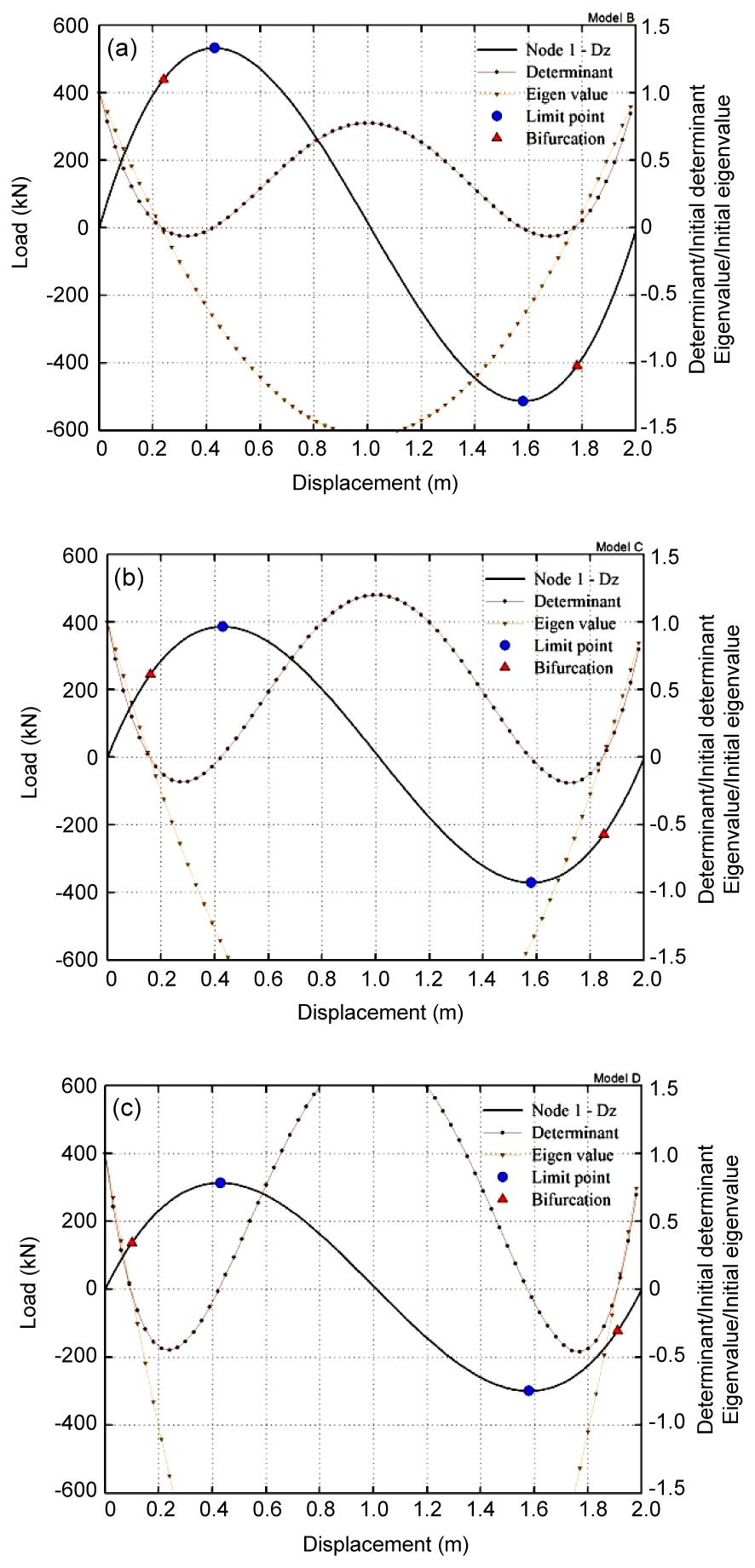Fig.3
Load-displacement curves of the two-free-nodes example (Model B, perfect sharp)
(a) A a/5; (b) A a/10; (c) A a/20

For all the three cases of Model B, the bifurcation point appeared before the limit point, which can be seen from the eigenvalue curve. The only difference is the decrease in load at the limit and bifurcation points due to the reduced cross-sectional area. All the equilibrium curves of cases 1, 2, and 3 changed their paths at the bifurcation point according to the initial conditions. Therefore, this study addressed only case 2 of Model B for the characteristics of the buckling load according to the bifurcation phenomenon and sensitive characteristics.

### 3.2.  Bifurcation behavior according to eigenmode and initial shape imperfection

The equilibrium path at the bifurcation point is very sensitive because of imperfection and real structures possess imperfection in various types. Examples include imperfection of nodes or foundation, violation of assumptions of materials or cross section, external load and shape, etc. Especially, the dome-shaped space truss, which manifests global unstable phenomenon in elastic range, is very sensitive to initial shape imperfection, and the buckling at one member or node extends to influence overall behavior of other members connected to it when there is a problem of instability for the case of the space truss connected to many members. Generally, the type of buckling taking place in the space truss is manifested as member buckling due to the buckling of a unit compression member, local buckling of the node snapping locally, and global buckling, in which the whole structure is buckled. However, the unstable phenomenon of space truss composed of many members such as the network dome or vault structural system has the aforementioned buckling behavior in complex influence, and it is not easy to explain the related phenomenon exactly. Thus, this study intends to investigate the characteristics of bifurcation in accordance with eigenmode and imperfect shape based on the example, in which the phenomenon appears relatively independent. The bifurcation buckling due to imperfection can consider buckling mode according to the eigenvalue analysis of the example.

The modes obtained by eigenvalue analysis of the example are shown in Fig. 4. The broken line represents the main member connected with the nodes number 7-2-1-3. The upper straight line is the shape projected to the X-Y plane and the bottom portrays the shape projected to the X-Z plane. The solid line representing an eigenmode along with the broken line is presented in the order of minimum eigenvalue. Although the eigenmode between the two models is similar in shape, it is a little different in order. Examining the characteristics of the eigenmode of the example, the first mode of asymmetric shape and the second mode of symmetric shape coincide in the two models, Models A and B (Case 2). However, the third mode of Model A is shown similar in shape to the fifth mode of Model B and is asymmetric as the first mode. Besides, the fourth and fifth modes of Model A are duplicated modes with the same eigenvalues and are asymmetric in shape coinciding with the third and fourth modes of Model B. The sensitivity to shape imperfection and bifurcation behavior for the aforementioned five eigenmodes were examined. Since the initial shape imperfection is generally considered for 0.2% of the maximum bottom diameter of a dome (Bulenda and Knippers, ), the imperfect shapes of 0.01%, 0.05%, 0.1%, and 0.3% were considered based on 2l, which is the short diameter in the example (Fig. 1).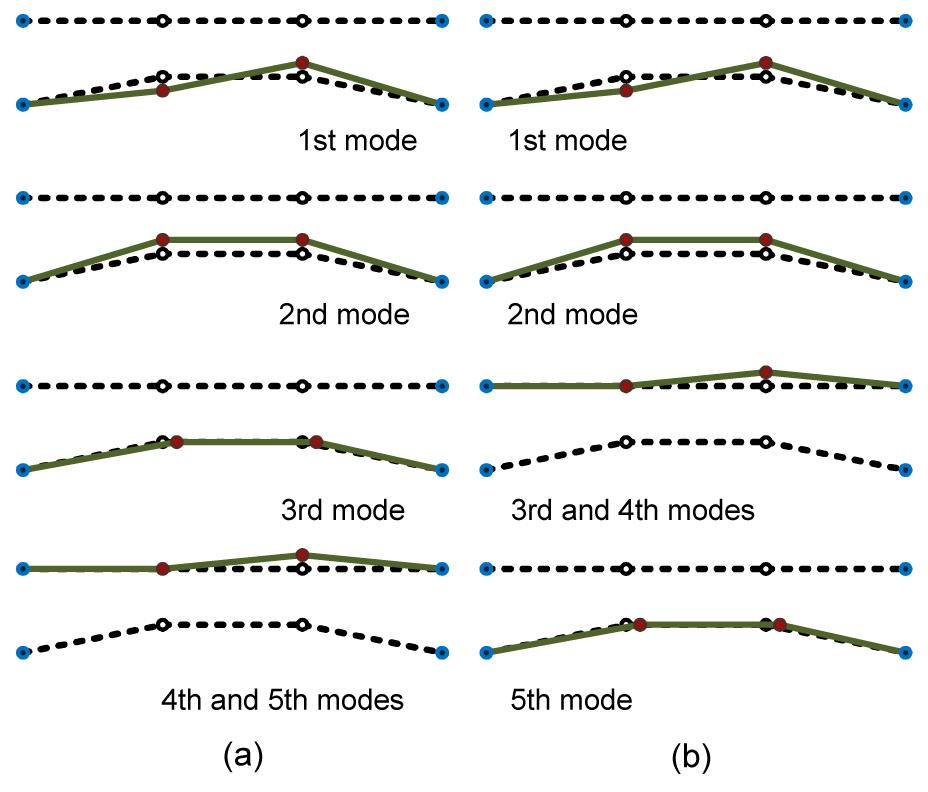Fig.4
Buckling modes of the two-free-nodes example
(a) Model A; (b) Model B (case 2)

The first mode of Models A and B was very sensitive when imperfection was 0.01% and behaves differently to perfect shape after the critical point as shown in Fig. 5.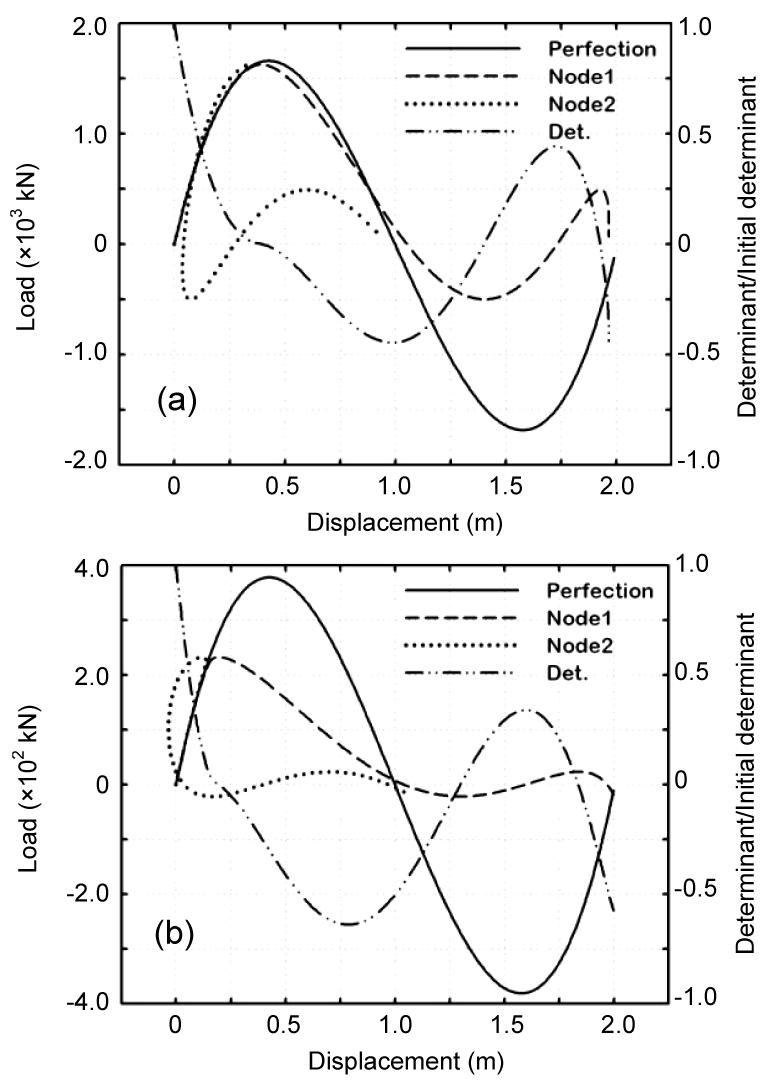Fig.5
Load-displacement curves of the two-free-nodes example (1st mode, 0.01% imperfection)
(a) Model A; (b) Model B (case 2)

The singular point shown as a one point chain line occurs at the turning point of the displacement, and the vertical displacement of two nodes progresses in different direction starting from the limit point for Model A and bifurcation point for Model B, respectively.

This pattern was also observed for the change at 0.3% imperfection (Fig. 6), which indicated large imperfection and resulted in the decrease in the load level at the critical point. The order of eigenvalue for the third mode of Model A and the fifth mode of Model B, which are eigenmodes of the second asymmetric shape, were very sensitive as the first mode (Fig. 7).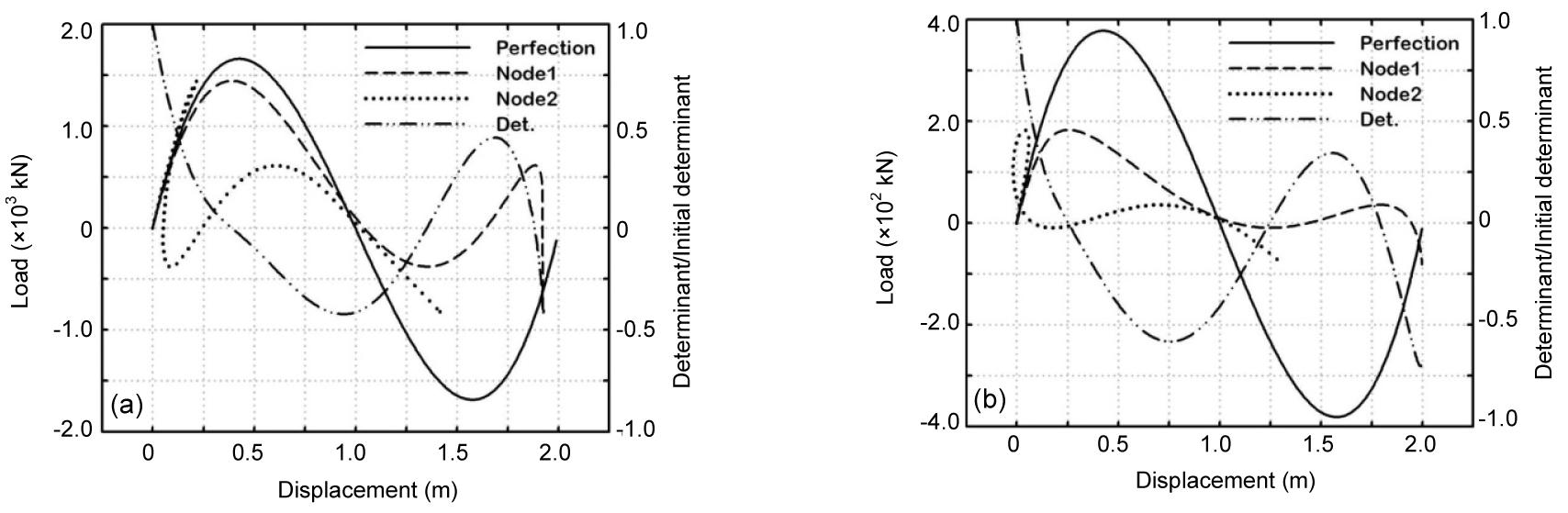Fig.6
Load-displacement curves of the two-free-nodes example (1st mode, 0.3% imperfection)
(a) Model A; (b) Model B (case 2)Fig.7
Load-displacement curves of the two-free-nodes example (0.01% imperfection)
(a) Model A (3rd mode); (b) Model B (case 2, 5th mode)

Although the decrease in the critical load level was not so large in comparison to the first mode, the decreasing pattern was the same. The second mode with symmetric shape of the two models behaved the same as the perfect shape even when the imperfection was 0.3% (Fig. 8), and the imperfection of the symmetric mode did not affect bifurcation. However, the limit load level was small in comparison to that of the perfect shape, and it could be predicted to decrease in proportion to the imperfection. The duplicated eigenmodes of main members with asymmetric shape in the out-of-plane direction had bifurcation behavior at 0.1% and 0.3% for Model A and Model B, respectively, and they were less sensitive, even if they had asymmetric shapes in comparison to other asymmetric shapes (Fig. 9).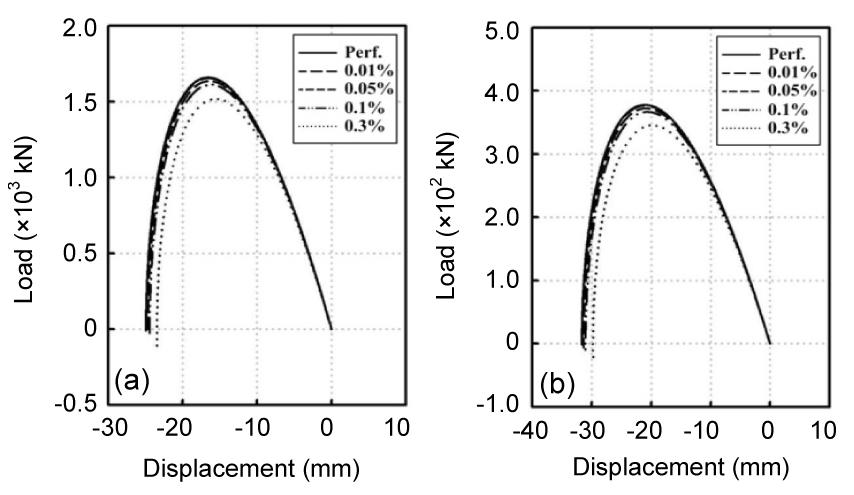Fig.8
Load-displacement curves of the two-free-nodes example (2nd mode, node 1-Dx )
(a) Model A; (b) Model B (case 2)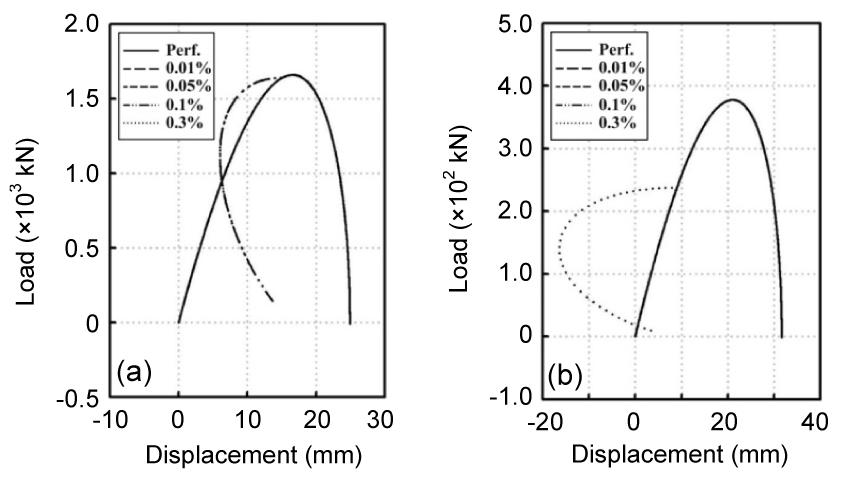Fig.9
Load-displacement curves of the two-free-nodes example (node 2-Dx )
(a) Model A (4th and 5th modes); (b) Model B (case 2, 3rd and 4th modes)

The two patterns were observed similarly in all cases regardless of Model A or Model B and were sensitive to the behavior of free nodes. The displaced position of the two free nodes was superposed at each incremental step in the plane of main members in order to explain this phenomenon as shown in Fig. 10. Explaining the two patterns of the figure, either the two nodes moved symmetrically as shown in Fig. 10a, or the vertical displacement moved asymmetrically at the critical point as shown in Fig. 10b. Perfect or imperfect shape with no bifurcation phenomenon will show the behavior in Fig. 10a, whereas bifurcation behavior due to an imperfect form appears in the pattern in Fig. 10b.Fig.10
Two patterns of unstable behavior of the two-free-nodes example
(a) Snap-through; (b) Bifurcation

If the X-Z plane, which is composed of only main member “a”, is considered for the analysis space, the two models will be the same as the model with four degrees of freedom (Fig. 11). In this case, the equilibrium matrix of the example will have one vector of U m=[0.2, 1.0, 0.2, −1.0]T, which is the in-extensional mechanism basis (Deng and Kwan, ; Pellegrino, ), and the shape will be similar to the eigenmode of the first asymmetric shape. Examining each composition (Table 2) of the first mode and basis vector of two models, Model B has the eigenmode, which is more advantageous in behaving similarly to the basis vector.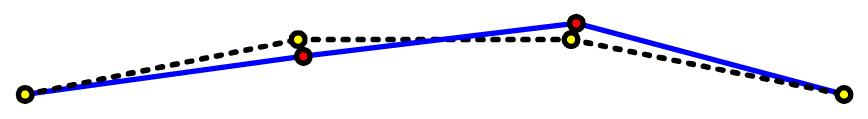Fig.11
In-extensional mechanism basis of the two-free-nodes example

#### Table 2

In-extensional mechanism basis and 1st eigenvector of Models A and B
 Node U m 1st eigenmode 1st eigenmode Model A Model B (case 2) 1 x 0.2 0.1098 0.185 y – 0.0 0.0 z 1.0 1.0 1.0 2 x 0.2 0.1098 0.185 y – 0.0 0.0 z −1.0 −1.0 −1.0 U m· v 1 2.0439 2.074

## 4.  Post-critical behavior and buckling load characteristics of the star dome

As shown in Model B of the two-free-nodes example, the first eigenmode is similar to the in-extensional mechanism basis and is sensitive to the initial conditions. The greater the number of nodes, the more complex the sensitivity and unstable conditions become, and the critical load may decrease due to the buckling of the nodes. In this section, the characteristics of unstable phenomenon and critical load according to the load mode will be discussed for the star dome, which is relatively simple although it has many nodes.

As shown in Fig. 12, the star dome consists of a total of 13 nodes and 24 members. All the nodes are located on the spherical surface with height, H and bottom radius, L.

Among the nodes, nodes 2–7 are on a ring that is away by L 1. The boundary condition is fixed until nodes 8–13, and the load acts vertically on nodes 1–7. To investigate the characteristics of the buckling load, which is reduced by nodal buckling, two parameters (i.e., rise-span ratio μ and load ratio R L) were considered. Here, μ is identical to the two-free-nodes example, and R L equals the load of node No. 2 (P r) divided by the load of node No. 1 (Pc), i.e., R L=P r/P c. In other words, R L>1 is the case where the ring load is large, and RL<1 is the case where the load of the center node is large.

### 4.1.  Eigenmode and post-critical behavior of the star dome

First of all, as shown in the two-free-nodes example, the equilibrium path of the star dome and the changes in the determinant and eigenvalue in each step will be examined.

The initial input data are shown in Table 3, which is the μ=0.102 model that was also used in (Hill et al., ). As a result of the eigenvalue analysis of the star dome, the eigenmode is shown in Fig. 13. In the first mode, the vertical element of node No. 1 is the largest, and the second mode has a large value at the ring node. Even though the eigenmode cannot represent the domes of all the shapes, the mode in this figure is a shape that can generally appear.

#### Table 3

Initial input data of the star dome (Hill et al., )
 Model H (m) H 1 (m) L (m) L 1 (m) μ Area (mm2) Star dome 1.0216 0.2 5.0 2.5 0.102 11.2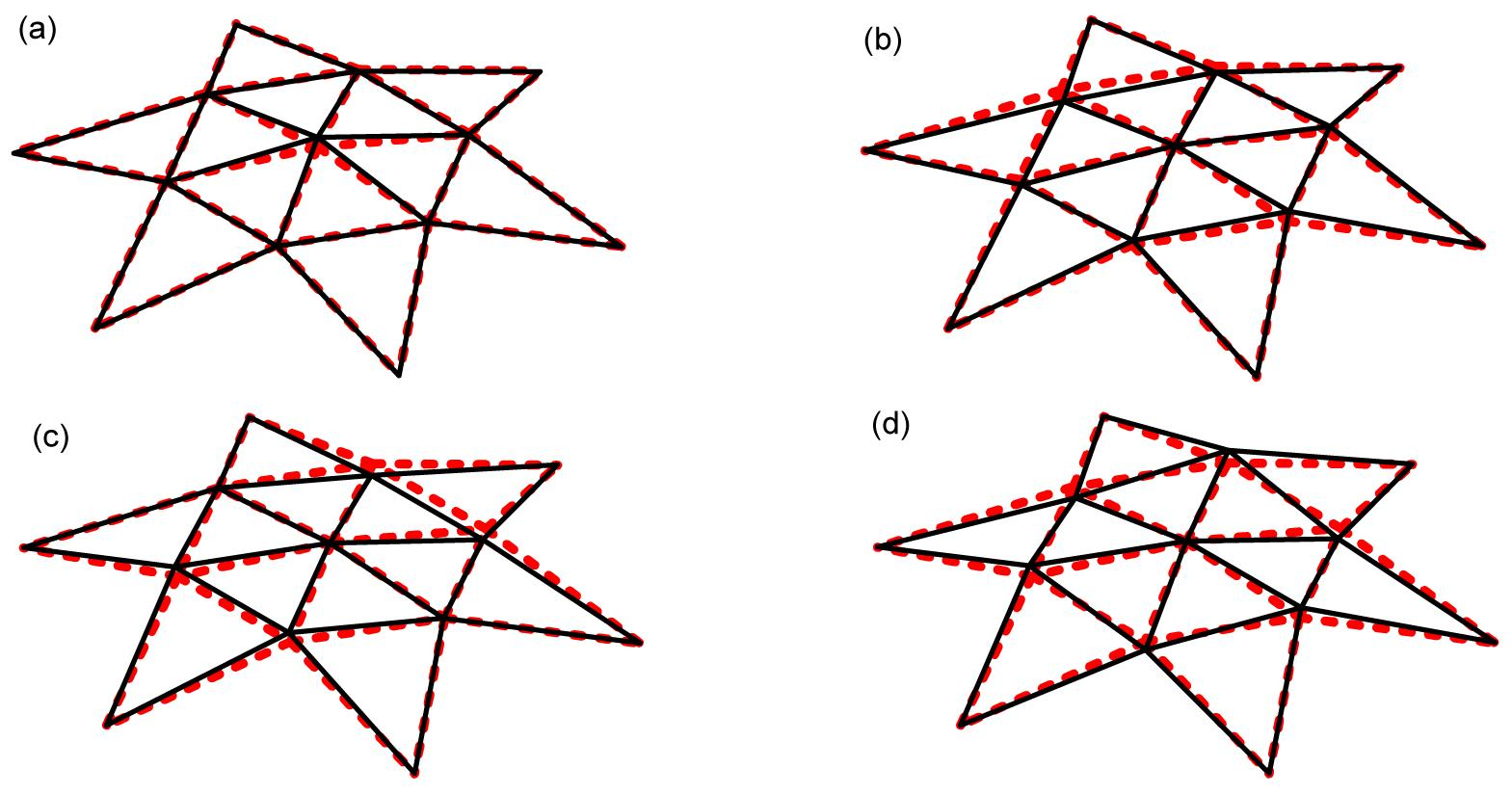Fig.13
Eigenmodes of the star dome
(a) 1st mode (b) 2nd mode; (c) 3rd mode (d) 4th mode

As a result of nonlinear analysis, the equilibrium path of the perfect-shape star dome is shown in Fig. 14, and the critical point on the equilibrium path appeared as a limit point with no change in the load level. As for the behavior after reaching the critical point in this figure, node No. 1 moves in the load direction, and node No. 2 moves in the opposite direction. In other words, the snapping appears at node No. 1, and the snap-back appears at node No. 2. As the load level decreases after reaching the critical point, dynamic snapping can be expected. According to Kim et al. (), the dynamic critical load is lower than the static critical load; thus, sudden nodal buckling will occur before the critical point is reached. As no bifurcation point occurs, however, no sensitive behavior due to an imperfect shape will occur under the same load condition. As shown in Fig. 15, the increase in the amount of imperfection due to the first eigenmode only decreases the buckling load, and no sensitive changes in the analysis results appear. Furthermore, a very large amount of imperfection results in a different phase of the structure, which does not need to be considered.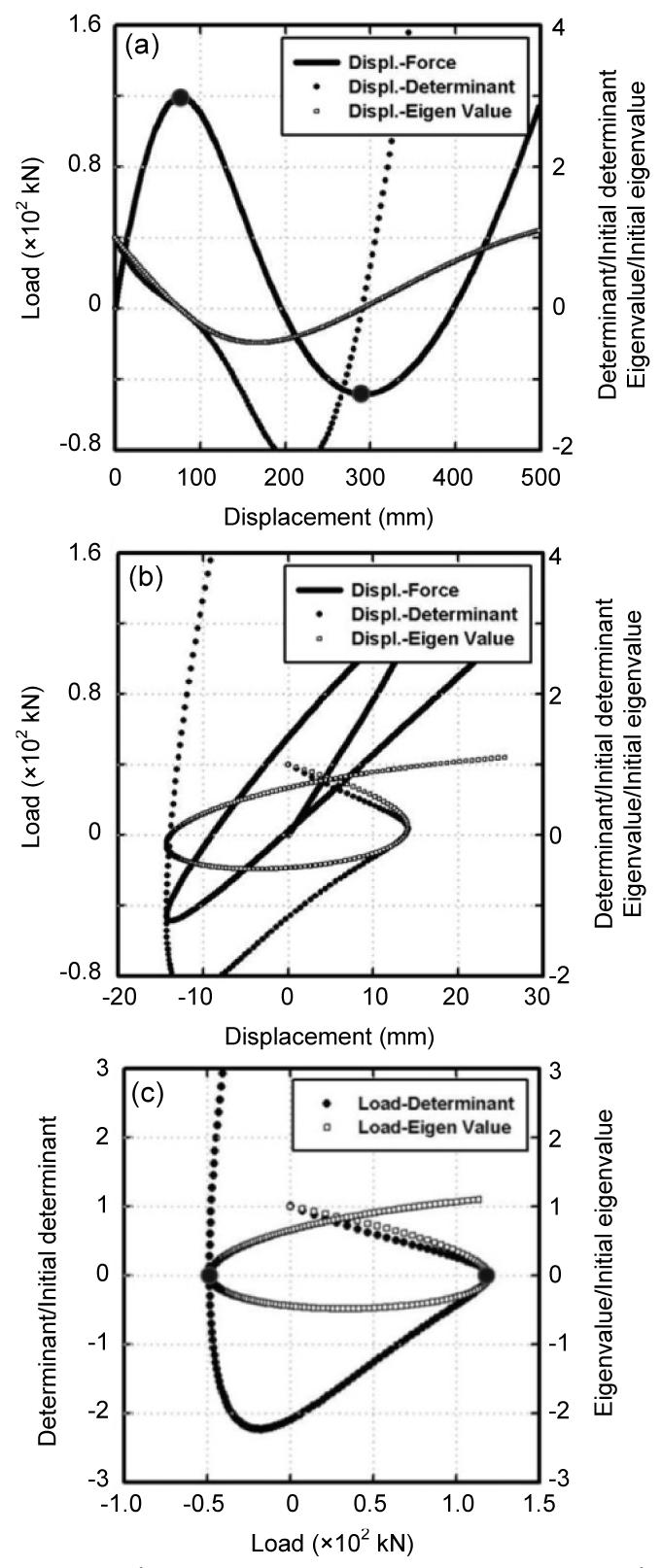Fig.14
Load-displacement curves and determinant and eigenvalue of the star dome
(a) Node 1-Dz ; (b) Node 2-Dz ; (c) Determinant and eigenvalue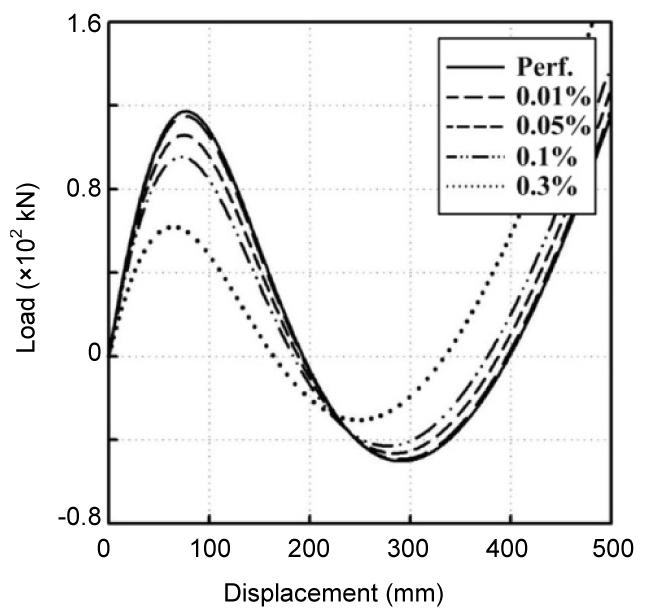Fig.15
Load-displacement curves of the star dome (1st eigenmode)

### 4.2.  Characteristics of the buckling load for the shape and the load mode

In the case of μ=0.102 in (Hill et al., ), R L=1; that is, the same load was applied at every node, and no bifurcation point occurred. The bifurcation points were observed according to the changes in μ and R L. The initial input data according to μ are shown in Table 4, and the load ratio R L changed from 0.6 to 1.7 in 0.1 intervals.

#### Table 4

Initial input data of the star dome in accordance with the rise-span ratio
 No. H (m) H 1 (m) L (m) L 1 (m) μ=H/(2L) 1 0.5 0.125 5.0 2.512 0.05 2 1.0 0.252 5.0 2.549 0.10 3 1.5 0.383 5.0 2.610 0.15 4 2.0 0.518 5.0 2.692 0.20 5 2.5 0.659 5.0 2.795 0.25 6 3.0 0.807 5.0 2.915 0.30 7 3.5 0.961 5.0 3.051 0.35 8 4.0 1.123 5.0 3.201 0.40 9 4.5 1.464 5.0 3.536 0.45

First, the analysis results for μ=0.15 are shown in Fig. 16, and the polygonal points on the load-displacement curve are the critical points. Here, Figs. 16a and 16b are the vertical displacement curves of node No. 1, and Figs. 16c and 16d are the vertical displacement curves of node No. 2. Furthermore, Figs. 16a and 16c are the analysis results for R L=0.6–1.0, and Figs. 16b and 16d are the analysis results for R L=1.1–1.7. As shown in Figs. 16a and 16c, the analysis results for R L=0.6–1.0 are similar to those for R L=0.102. In other words, no bifurcation point appeared before the limit point, and the two nodes progressed in different directions after reaching the critical point. In the case of R L=1.1–1.7, however, a bifurcation point occurred before the limit point, and the two nodes progressed in the same directions as those before the critical point. Considering the moving directions of the two nodes, snapping of the total structure is expected in the latter case, and the load level at the bifurcation point becomes the buckling load. Judging from the bifurcation point, the bifurcation phenomenon will appear according to the initial conditions, and the bifurcation path will be similar to that of the two-free-nodes example.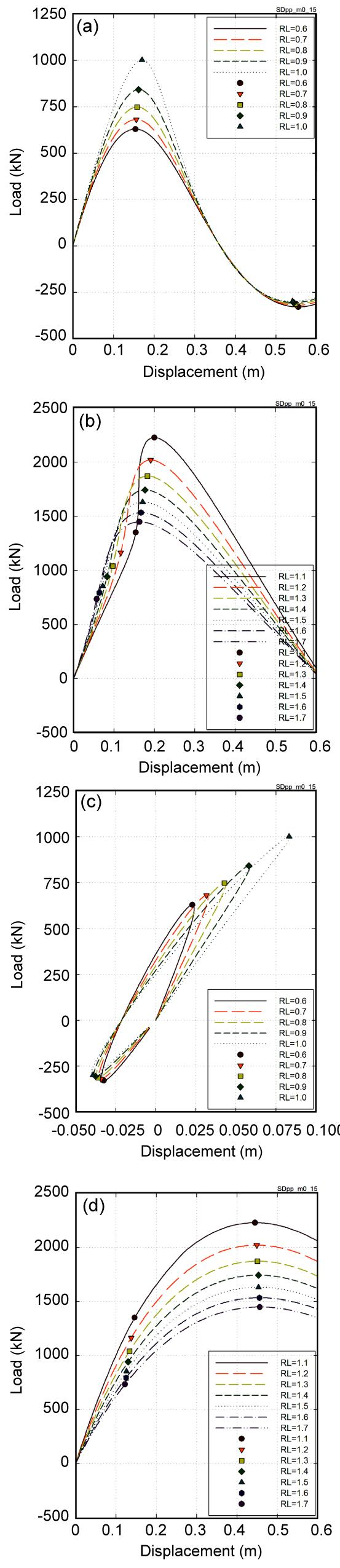Fig.16
Load-displacement curves of the star dome (μ=0.15)
(a) Node 1-Dz (R L=0.6–1.0); (b) Node 1-Dz (R L=1.1–1.7); (c) Node 2-Dz (R L=0.6–1.0); (d) Node 2-Dz (R L=1.1–1.7)

Besides, the results for μ=0.35 show the same characteristics (Fig. 17). R L at the interface between the two patterns, however, increased along with the shape parameter μ.Fig.17
Load-displacement curves of the star dome (μ=0.35)
(a) Node 1-Dz (R L=0.6–1.4); (b) Node 1-Dz (R L=1.5–1.7); (c) Node 2-Dz (R L=0.6–1.4); (d) Node 2-Dz (R L=1.5–1.7)

Next, the bifurcation point and critical load at each μ and R L are shown in Fig. 18. The buckling load (P cr) was compared to the limit load (P 0) of R L=1. R L at which the bifurcation point began to appear gradually increased as μ increased, but no bifurcation point appeared at μ=0.45. Furthermore, in the case of R L, where no bifurcation point appeared, the critical load increased as the R L increased, but the critical load gradually decreased in the bifurcation model. Based on the results of the example, when μ is larger at the bifurcation point, or when the shape is higher, the snapping of the center node will begin first.Fig.18
Buckling load ratio of the limit point and bifurcation of the star dome
(a) μ=0.05; (b) μ=0.1; (c) μ=0.15; (d) μ=0.2; (e) μ=0.25; (f) μ=0.3; (g) μ=0.35; (h) μ=0.4; (i) μ=0.45

In the case of R L, however, which contains the bifurcation point, a bifurcation behavior sensitive to the initial conditions will appear, which is similar to the two-free-nodes example. Here, the buckling load of the structure for which a bifurcation point occurs is about 50%–60% of the load level at the limit point, as shown in Fig. 19.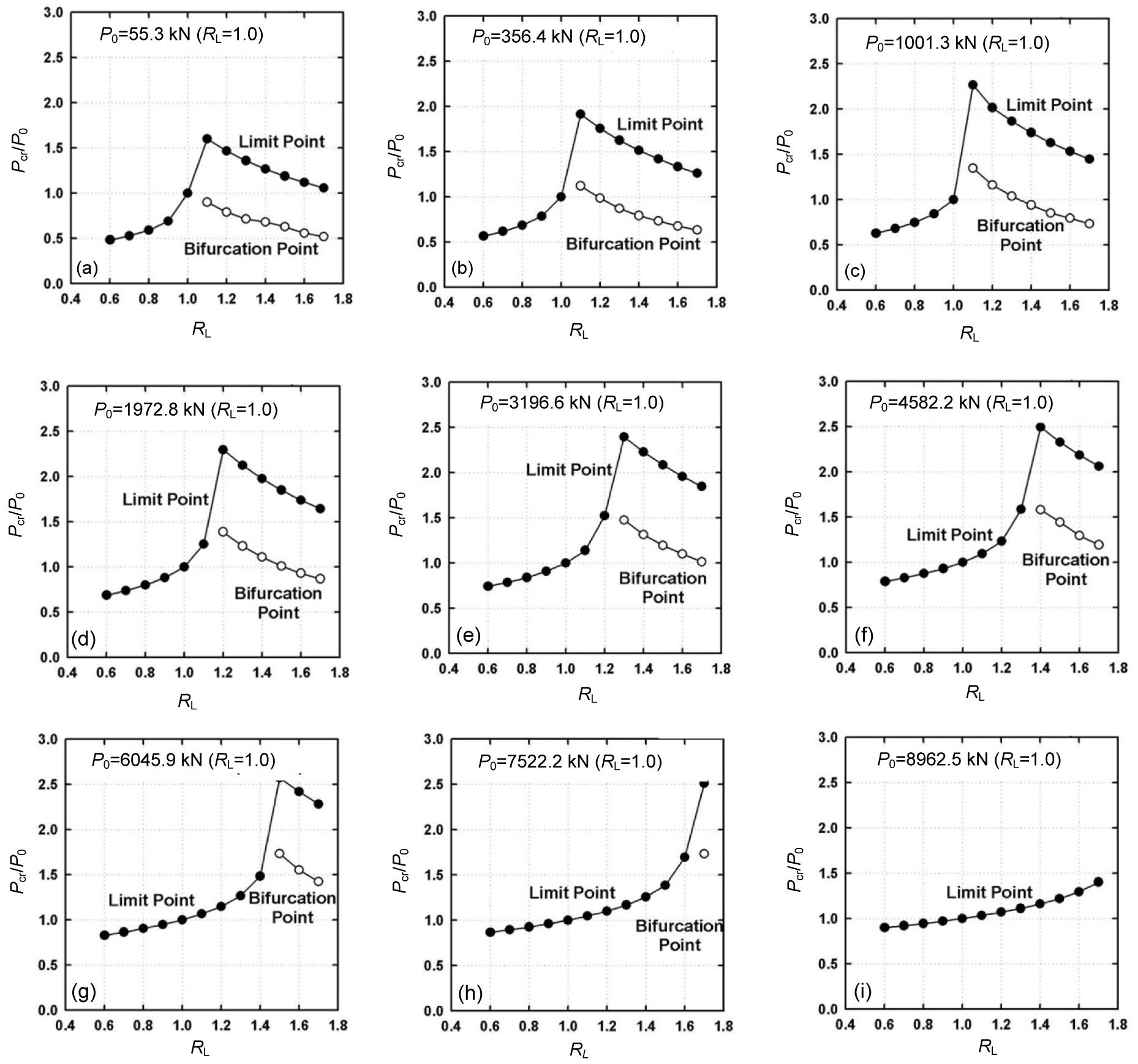Fig.19
Critical load ratio of bifurcation to the limit point of the star dome

## 5.  Conclusions

In this study, the determination of the unstable points and buckling load characteristics for a space truss sensitive to initial shape imperfection was investigated. To precisely examine the sensitivity according to the eigenmode and the bifurcation phenomenon, a two-free-nodes example, a shallow steel space truss, was adopted, and the star dome was also adopted to analyze the critical-load characteristics according to the node and global buckling. The sensitivity according to the eigenmode of the two-free-nodes example resulted in a change in the equilibrium path at the bifurcation point and to a great decrease in buckling load despite the slightly imperfect shape. The two buckling patterns that appeared in this example varied depending on the occurrence of bifurcation behavior, and the asymmetric eigenmode had the greatest impact on the unstable behavior at the critical point due to the imperfect shape. In particular, the first eigenmode was similar to the in-extensional mechanism basis of the simplified model. For the star dome, the greater the value of μ was, or the higher the shape was, the more easily nodal buckling occurred rather than global buckling, and the structure showed unstable behavior. Besides, the greater the load ratio R L was, the more likely it was that the bifurcation point appeared on the equilibrium path, and at this point, the buckling load level was about 50%–60% of the load level at the limit point.

* Project (No. 2012-0005418) supported by the Basic Science Research Program through the National Research Foundation of Korea (NRF) funded by the Ministry of Education, Science and Technology

## ReferencesAbedi, K., Parke, G.A.R., 1991. Progressive collapse of single-layer braced domes. International Journal of Space Structures, 11(3):291-306.Bulenda, T., Knippers, J., 2001. Stability of grid shells. Computers and Structures, 79(12):1161-1174.Chan, S.L., Zhou, Z.H., 1995. Second-order elastic analysis of frames using single imperfect element per member. Journal of Structural Engineering, 121(6):939-945.Choong, K.K., Hangai, Y., 1993. Review on methods of bifurcation analysis for geometrically nonlinear structures. Bulletin of the International Association for Shell and Spatial Structures, 34(112):133-149.Choong, K.K., Kim, J.Y., 2001. A numerical strategy for computing the stability boundaries for multi-loading systems by using generalized inverse and continuation method. Engineering Structures, 23(6):715-724.Deng, H., Kwan, A.S.K., 2005. Unified classification of stability of pin-jointed bar assemblies. International Journal of Solids and Structures, 42(15):4393-4413.El-Sheikh, A., 1998. Design of space truss structures. Structural Engineering and Mechanics, 6(2):185-200.Fan, F., Yan, J., Cao, Z., 2010. Calculation Methods of Stability of Single-Layer Reticulated Domes Considering the Effect of Initial Bending of Members. , Proceedings of the IASS Symposium, Shanghai, 339-347. :339-347.Fan, F., Ma, H., Cao, Z., Shen, S., 2011. A new classification system for the joints used in lattice shells. Thin-Walled Structures, 49(12):1544-1553.Gao, B., Lu, Q., Dong, S., 2003. Geometrical nonlinear stability analyses of cable-truss domes. Journal of Zhejiang University-SCIENCE, 4(3):317-323.Hill, C.D., Blandford, G.E., Wang, S.T., 1989. Post-bucking analysis of steel space trusses. Journal of Structural Engineering, 115(4):900-919.Huseyin, K., 1973. The multi-parameter perturbation technique for the analysis of nonlinear system. International Journal of Non-Linear Mechanics, 8(5):431-443.Hwang, K.J., Knippers, J., 2010. Stability of Single Layered Grid Shells with Various Connectors. , Proceedings of the ICSA Guimaraes, Portugal, 581-588. :581-588.Kato, S., Mutoh, I., Shomura, M., 1994. Effect on joint rigidity on buckling strength of single layer lattice dome. Bulletin of the International Association for Shell and Spatial Structures, 35(115):101-109.Kato, S., Yamashita, T., Nakazawa, S., Kim, Y., Fujibayashi, A., 2007. Analysis based evaluation for buckling loads of two-way elliptic paraboloidal single layer lattice domes. Journal of Constructional Steel Research, 63(9):1219-1227.Kato, S., Nakazawa, S., Nobe, K., 2010. Semi-Probabilistic Evaluation of Buckling Strength of Two-Way Single Layer Lattice Dome. , Proceedings of the IASS Symposium, Shanghai, 384-395. :384-395.Kim, S.D., Kang, M.M., Kwun, T.J., Hangai, Y., 1997. Dynamic instability of shell-like shallow trusses considering damping. Computers and Structures, 64(1-4):481-489.Lopez, A., Puente, I., Serna, M.A., 2007. Numerical model and experimental tests on single-layer latticed domes with semi-rigid joints. Computers and Structures, 85(7-8):360-374.Ma, H., Fan, F., Shen, S., 2009. Numerical Parametric Investigation of Single-Layer Latticed Domes with Semi-Rigid Joints. , Proceedings of the IASS Symposium, Valencia, 99-110. :99-110.Mang, H.A., Schranz, C., Mackenzie-Helnwein, P., 2006. Conversion from imperfection-sensitive into imperfection-insensitive elastic structures I: Theory. Computer Methods in Applied Mechanics and Engineering, 195(13-16):1422-1457.Pellegrino, S., 1993. Structural computations with the singular value decomposition of the equilibrium matrix. International Journal of Solids and Structures, 30(21):3025-3035.Schranz, C., Krenn, B., Mang, H.A., 2006. Conversion from imperfection-sensitive into imperfection-insensitive elastic structures II: Numerical investigation. Computer Methods in Applied Mechanics and Engineering, 195(13-16):1458-1479.Shon, S.D., Kim, S.D., Kang, M.M., 2002. Nonlinear Instability Analysis of Framed Space Structures with Semi-rigid Joints. , Proceedings of the IASS Symposium, Warsaw, 422-427. :422-427.Steinboeck, A., Jia, X., Hoefinger, G., Mang, H.A., 2008. Conditions for symmetric, antisymmetric, and zero-stiffness bifurcation in view of imperfection sensitivity and insensitivity. Computer Methods in Applied Mechanics and Engineering, 197(45-48):3623-3626.Thompson, J.M.T., Hunt, G.W., 1983. On the buckling and imperfection-sensitivity of arches with and without prestress. International Journal of Solids and Structures, 19(5):445-459.Uros, M., Lazarevic, D., Gidak, P., 2011. Interaction of Member and Node Instability in the Single Layer Reticulated Shell. , Proceedings of the IABSE-IASS Symposium, London, 168:168Yamada, S., Matsumoto, Y., Sakamoto, A., 2011. Design Estimation Method of Buckling Load and the Associated Mode for Single Layer Lattice Dome Roof with Square Plan. , Proceedings of the IABSE-IASS Symposium, London, 72:72You, K.H., Kim, M.S., Cho, B.W., 2010. Instability Characteristic of Space Frames with Various Networks. , Proceedings of the IASS Symposium, Shanghai, 1505-1516. :1505-1516.